# Inverse element facts for kids

Kids Encyclopedia Facts

The inverse element is the opposite of a number or equation. The inverses of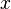$x$ in addition is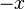$-x$ (called the additive inverse), and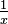$\tfrac{1}{x}$ or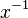$x^{-1}$ in multiplication (called the multiplicative inverse, or reciprocal).

In general, given an element g in a group S equipped with an operation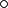$\circ$, an inverse element of g is an element g* such that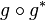$g \circ g^*$ and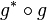$g^* \circ g$ are both equal to the identity element (of the group). Other examples of inverse include inverse function and matrix inverse.

## Related pages

• Field (mathematics), a set equipped with additive inverses and multiplicative inverses (except for zero)In Spanish: Elemento simétrico para niños